# Selina solutions for Concise Mathematics Class 7 ICSE chapter 19 - Congruency: Congruent Triangles [Latest edition]

#### Chapters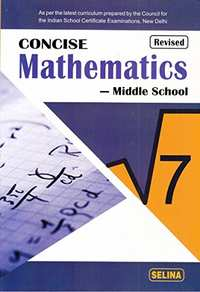## Chapter 19: Congruency: Congruent Triangles

Exercise 19
Exercise 19

### Selina solutions for Concise Mathematics Class 7 ICSE Chapter 19 Congruency: Congruent Triangles Exercise 19

Exercise 19 | Q 1.1

State, whether the pairs of triangles given in the following figures are congruent or not: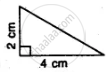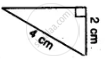Exercise 19 | Q 1.2

State, whether the pairs of triangles given in the following figures are congruent or not: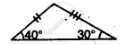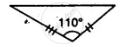Exercise 19 | Q 1.3

State, whether the pairs of triangles given in the following figures are congruent or not: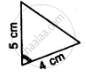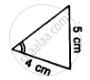Exercise 19 | Q 1.4

State, whether the pairs of triangles given in the following figures are congruent or not: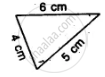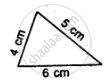Exercise 19 | Q 1.5

State, whether the pairs of triangles given in the following figures are congruent or not: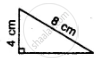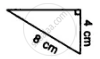Exercise 19 | Q 1.6

State, whether the pairs of triangles given in the following figures are congruent or not: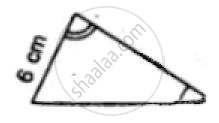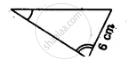Exercise 19 | Q 1.7

State, whether the pairs of triangles given in the following figures are congruent or not:

Δ ABC in which AB = 2 cm, BC = 3.5 cm and ∠C = 80° and Δ DEF in which DE = 2 cm, DF = 3.5 cm and ∠D = 80°.

Exercise 19 | Q 2

In the given figure, prove that: ∆ ABD ≅ ∆ ACD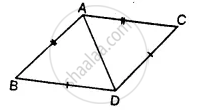Exercise 19 | Q 3

Prove that:
(i) ∆ ABC ≅ ∆ ADC
(ii) ∠B = ∠D
(iii) AC bisects angle DCB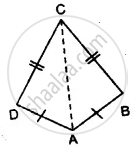Exercise 19 | Q 4

Prove that:
(i) ∆ ABD ≅ ∆ ACD
(ii) ∠B = ∠C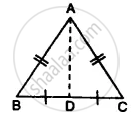Exercise 19 | Q 5

In the given figure, prove that:
(i) ∆ ACB ≅ ∆ ECD
(ii) AB = ED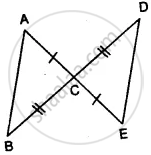Exercise 19 | Q 6

Prove that:
(i) ∆ ABC ≅ ∆ ADC
(ii) ∠B = ∠D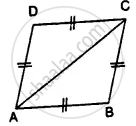Exercise 19 | Q 7

In the given figure, prove that: BD = BC.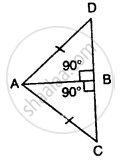Exercise 19 | Q 8

In the given figure ;
∠1 = ∠2 and AB = AC.
Prove that:
(i) ∠B = ∠C
(ii) BD = DC
(iii) AD is perpendicular to BC.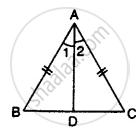Exercise 19 | Q 9

In the given figure prove that:
(i) PQ = RS
(ii) PS = QR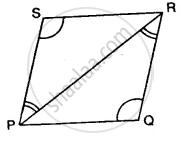Exercise 19 | Q 10

(i) ∆ XYZ ≅ ∆ XPZ
(ii) YZ = PZ
(iii) ∠YXZ = ∠PXZ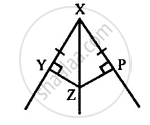Exercise 19 | Q 11

In the given figure, prove that:
(i) ∆ ABC ≅ ∆ DCB
(ii) AC = DB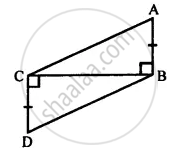Exercise 19 | Q 12

In the given figure, prove that:
(i) ∆ AOD ≅ ∆ BOC
(iv) ∆ ADB ≅ ∆ BCA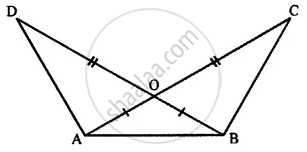Exercise 19 | Q 13

ABC is an equilateral triangle, AD and BE are perpendiculars to BC and AC respectively. Prove that:
(ii) BD = CE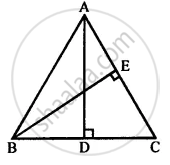Exercise 19 | Q 14

Use the information given in the following figure to prove triangles ABD and CBD are congruent. Also, find the values of x and y.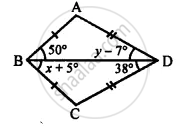Exercise 19 | Q 15

The given figure shows a triangle ABC in which AD is perpendicular to side BC and BD = CD. Prove that:
(i) ∆ ABD ≅ ∆ ACD
(ii) AB = AC
(iii) ∠B = ∠C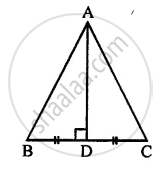## Chapter 19: Congruency: Congruent Triangles

Exercise 19## Selina solutions for Concise Mathematics Class 7 ICSE chapter 19 - Congruency: Congruent Triangles

Selina solutions for Concise Mathematics Class 7 ICSE chapter 19 (Congruency: Congruent Triangles) include all questions with solution and detail explanation. This will clear students doubts about any question and improve application skills while preparing for board exams. The detailed, step-by-step solutions will help you understand the concepts better and clear your confusions, if any. Shaalaa.com has the CISCE Concise Mathematics Class 7 ICSE solutions in a manner that help students grasp basic concepts better and faster.

Further, we at Shaalaa.com provide such solutions so that students can prepare for written exams. Selina textbook solutions can be a core help for self-study and acts as a perfect self-help guidance for students.

Concepts covered in Concise Mathematics Class 7 ICSE chapter 19 Congruency: Congruent Triangles are Concept for Congruence Through Superimposition, Extend Congruence to Simple Geometrical Shapes E.G. Triangles, Circles., Criteria for Congruence of Triangles, Congruence of Triangles.

Using Selina Class 7 solutions Congruency: Congruent Triangles exercise by students are an easy way to prepare for the exams, as they involve solutions arranged chapter-wise also page wise. The questions involved in Selina Solutions are important questions that can be asked in the final exam. Maximum students of CISCE Class 7 prefer Selina Textbook Solutions to score more in exam.

Get the free view of chapter 19 Congruency: Congruent Triangles Class 7 extra questions for Concise Mathematics Class 7 ICSE and can use Shaalaa.com to keep it handy for your exam preparation